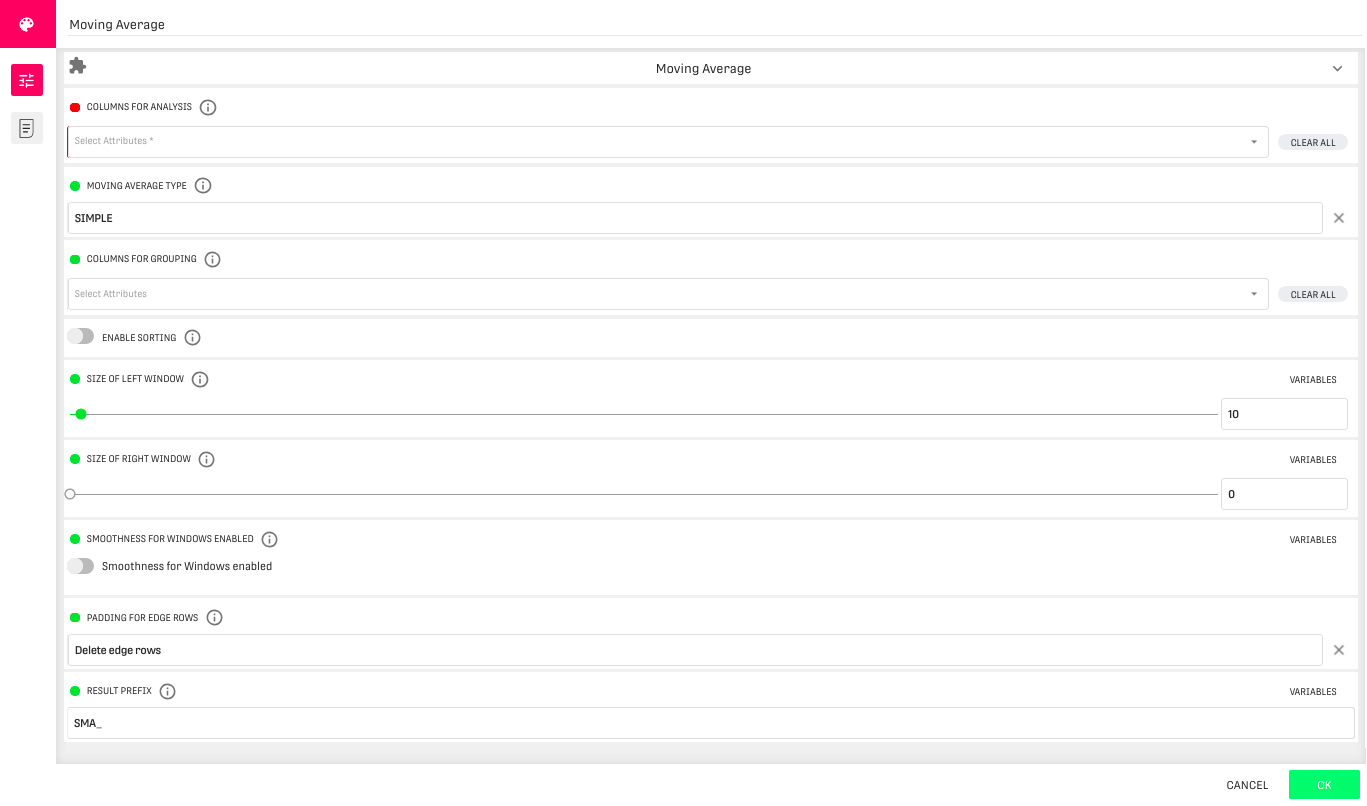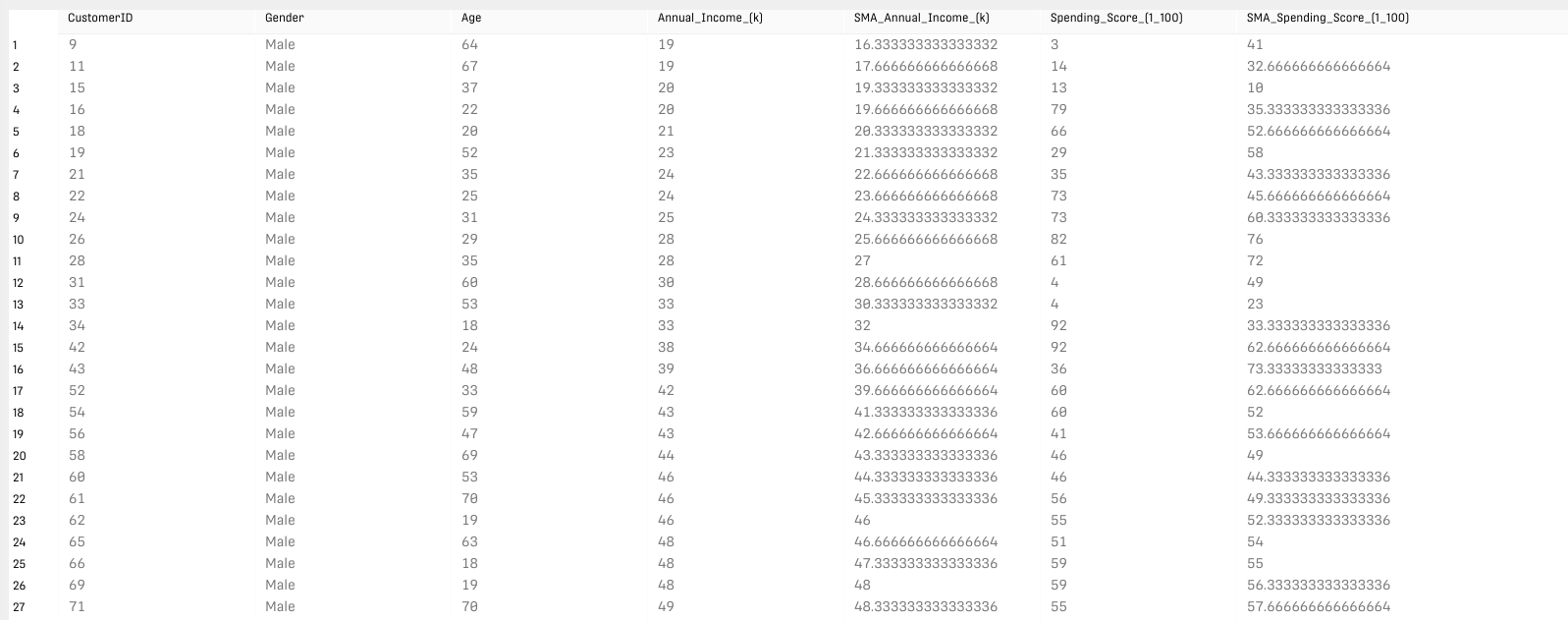# Moving Average Processor

Modified on Tue, 30 Nov 2021 at 04:47 PM

# Overview

The Moving Average Processor is used for analyzing data by creating series of averages of different subsets of the dataset. In general, a moving average (MA) is a widely used indicator in technical analysis that helps smooth out price action by filtering out the “noise” from random short-term price fluctuations.

# Input

The input dataset should contain at least one numerical column, for which the moving average should be calculated.

# ConfigurationColumns for Analysis: Columns which will be analysed using the chosen moving average type.

Moving Average Types:

• Simple: The simple algorithm calculates the average of values in one window.
• triangular: The triangular algorithm calculates the average of all previously calculated simple moving average values in one window.
• weighted: The weighted algorithm calculates the average of all values in one window, but puts weights to the values depending on the distance to the position of the actual line.

Columns for Grouping: Column, which is used for grouping the data before any moving average calculations are done.

If this is set it is mandatory to also specify at least one sorting column.

Enable Sorting: Sorts the data by the specified columns prior to calculation.

Size of Left Window: Maximum size of the left window from the current element. The left and right window will sum up to the used window.

Size of Right Window: Maximum size of the right window from the current element. The left and right window will sum up to the used window.

Smoothness for Windows Enabled: Smoothness means: E.g. if the moving average has to be calculated for the second element with a symmetric window, this might not be possible for a window bigger than 3. Hence the window adjusts to its biggest possible size of 3 elements, if smoothness is enabled.

Padding for Edge Rows: Specifies how edge rows for which no moving average can be calculated are treated in the result.

Result Prefix: Appended to the original column names used for the moving average.

# Output

The processor returns a table with the same columns as the input dataset along with an additional column for each option selected in the first configuration field. The output dataset will contain less rows than the input data depending on the chosen window size.

# Example

In the following example, we use a dataset of mall customers. The moving average is calculated using both the annual income and spending score columns for each gender ordered by age.

## ResultOur original data has 200 entries. if we use a left window of size 2 and a right window of size 0 with a moving average of type simple, we will have 198 entries as a result (the algorithm ignores the first two entries). If we group the data by gender, the first two entries of each gender will be ignored. Therefore the number of entries in the output is 196.

# Related Articles

Grouped Root Mean Square Processor

Grouped FFT Computation Processor